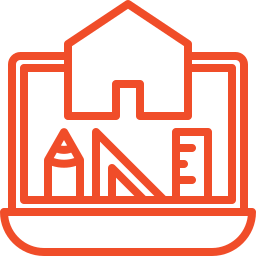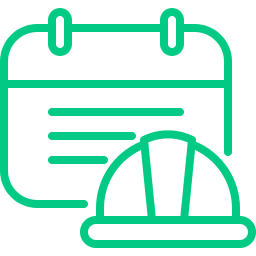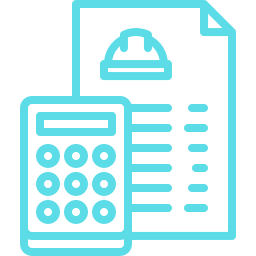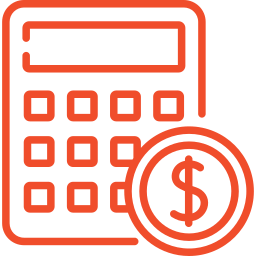# Construction Calculators

Our online construction calculators were designed by BuildBook to help builders and remodelers save time and money by providing a quick and free way to calculate the most critical parts of the job.## Construction Costs

### Construction Software Cost CalculatorCalculate and compare the cost of different construction management software platforms

## Construction Costs

### Construction Timeline CalculatorCalculate the length of a construction project in days, weeks, and months

## Construction Financials

### Construction Estimate ROICalculate the time and revenue won and lost each year generating estimates

## Construction Measurements

### Drywall CalculatorCalculate the quantity of drywall sheets, joint compound, tape, and screws you'll need for a project

## Construction Financials

### Hourly Rate CalculatorDetermine how much to charge per hour as a general contractor, subcontractor, or handyman

## Construction Measurements

### Brick CalculatorCalculate the quantity and total cost of concrete blocks needed based on the dimensions of a wall.

## Construction Financials

### Net Profit CalculatorCalculate how much construction revenue you need to achieve your net profit goal

## Construction Measurements

### Framing CalculatorQuickly determine the number of studs you need and the total cost to build a wall

## Construction Costs

### Construction CostsCalculate your total construction costs based on line items, rates, and quantities

## Construction Measurements

### Square Footage CalculatorFind the total square footage of a square, rectangular, circular, or triangular area

## Construction Measurements

### Concrete CalculatorCalculate the amount of concrete needed in cubic feet, yards, and bags for a concrete slab

## Construction Costs

### Labor Cost CalculatorFind the total and hourly labor cost with total labor burden for any construction project

## Construction Measurements

### Board Foot CalculatorQuickly estimate the total board feet and the cost per foot of your lumber for a project

## Construction Financials

### Profit Margin CalculatorDiscover what to charge and find your profit margin based on your markup percentage

## Construction Financials

### Markup CalculatorDiscover what to charge and find your markup percentage based on your target profit margin

## Win better projects by making a better first impression

(Yep, it’s totally free. No fine print other than what you're reading now.)